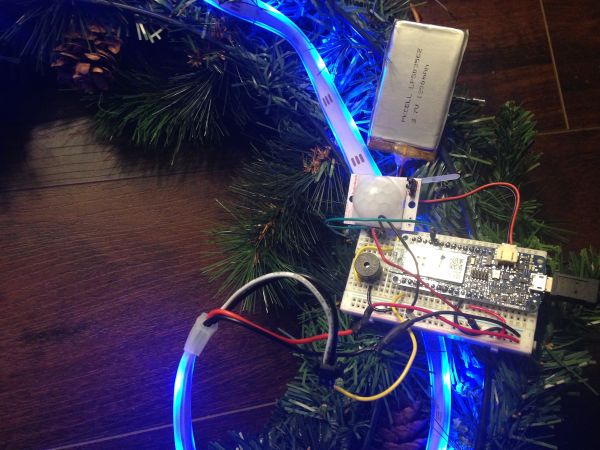# Reverse-engineering the ALU of 8008 microprocessor

A computer’s arithmetic-logic unit (ALU) is the heart of the processor, performing arithmetic and logic operations on data. If you’ve studied digital logic, you’ve probably learned how to combine simple binary adder circuits to build an ALU. However, the 8008’s ALU uses clever logic circuits that can perform multiple operations efficiently. And unlike most 1970’s microprocessors, the 8008 uses a complex carry-lookahead circuit to increase its performance.The 8008 was Intel’s first 8-bit microprocessor, introduced 45 years ago.1 While primitive by today’s standards, the 8008 is historically important because it essentially started the microprocessor revolution and is the ancestor of the x86 processor family that you are probably using right now.2 I recently took some die photos of the 8008, which I described earlier. In this article, I reverse-engineer the 8008’s ALU circuits from these die photos and explain how the ALU functions.

# Inside the 8008 chip

The image below shows the 8008’s tiny silicon die, highly magnified. Around the outside of the die, you can see the 18 wires connecting the die to the chip’s external pins. The rest of the chip contains the chip’s circuitry, built from about 3500 tiny transistors (yellow) connected by a metal wiring layer (white).

Many parts of the chip work together to perform an arithmetic operation. First, two values are copied from the registers (on the right side of the chip) to the ALU’s temporary registers (left side of the chip) via the 8-bit data bus. The ALU computes the result, which is stored back into the accumulator register via the data bus. (Note that the data bus splits and goes around both sides of the ALU to simplify routing.) The carry lookahead circuit generates the carry bits for the sum in parallel for higher performance.3 This is all controlled by the instruction decode logic in the center of the chip that examines each machine instruction and generates signals that control the ALU (and other parts of the chip).

# The Arithmetic-Logic Unit

The 8008’s ALU implements four functions: Sum, AND, XOR and OR. The Sum operation adds two 8-bit numbers. The remaining three operations are standard Boolean logic operations. The AND operation sets an output bit if the bit is set in the first AND the second number. OR checks if a bit is set in the first OR the second number (or both). XOR (exclusive-or) checks if a bit is set in the first OR the second number (but not both).

The concept of carries during addition is a key part of the ALU. Binary addition in a processor is similar to grade-school long addition, except with binary numbers instead of decimal. Starting at the right, each column of two numbers is added and there can be a carry to the next column. Thus, in each column, the ALU adds two bits as well as a carry bit.